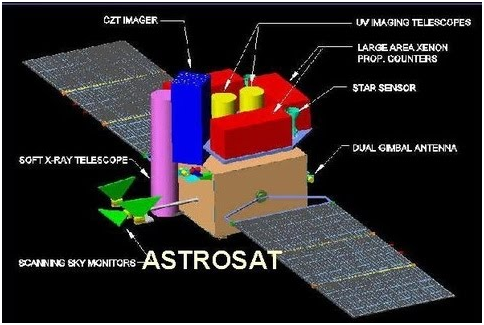# ON FIXED POINT THEOREM AND ITS APPLICATIONS IN DIFFERENT METRIC SPACE

Fixed point theory occupies a prominent place in the study of metric spaces. One of the important questions that may arise in this connection is whether metric spaces really provide enough space for this theory or not. Recently, in Huang and Zhang (2007), the authors rather implied that the answer is no. Actually, they introduced the notion of cone metric space, and gave an example of a function which is a contraction in the category of cone metric spaces but not a contraction if considered over metric spaces and hence, by proving a fixed point theorem in cone metric spaces, ensured that this map must have a unique fixed point. After that a series of articles about cone metric spaces started to appear. Some of those articles dealt with fixed point theorems in those spaces, specially in complete ones, and others dealt with the structure of the spaces themselves.
On the other hand Cone metric spaces were introduced in Huang and Zhang (2007). The authors there described convergence in cone metric spaces, and introduced completeness. Then they proved some fixed point theorems of contractive mappings on cone metric spaces, where they also provided an example of a contractive mapping on a cone metric space with E = R2 and P = (x, y) ∈ R2: x, y ≥ 0. However this mapping is not contractive in the Euclidean metric space. Then recently, in (Ili and Rako, 2008; Abbas, and Jungck, 2008) some common fixed point theorems were proved for maps on cone metric spaces. In this paper we introduce some basic topological concepts and definitions in cone metric spaces. Actually, we prove that every cone metric space is a topological space. Then we generalize the concepts diametrical contractivity and asymptotical diametrical contractivity to cone metric spaces. Finally we obtain some fixed point theorems for such mappings. By this we generalize some results from Xu(2004) to cone metric spaces.

Akanksha Shukla